# Each row of the table below describes an aqueous solution at about 25 °C. Complete the...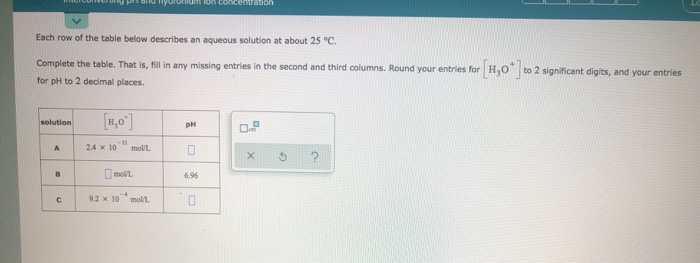Each row of the table below describes an aqueous solution at about 25 °C. Complete the table. That is, fill in any missing entries in the second and third columns. Round your entries for H,O" to 2 significant digits, and your entries for pH to 2 decimal places solution 0. ,0) 24 x 10" mall X 5 ? 9.2 x 10 moll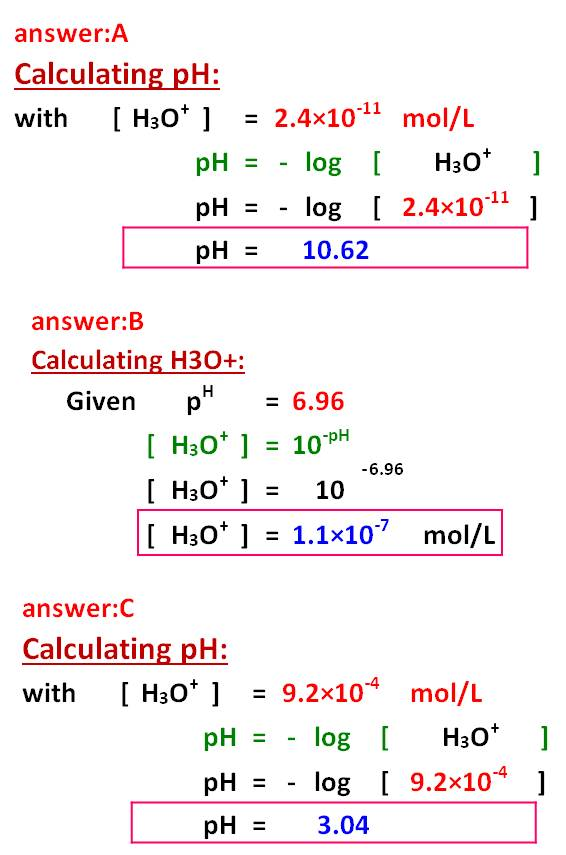#### Earn Coin

Coins can be redeemed for fabulous gifts.

Similar Homework Help Questions
• ### Each row of the table below describes an aqueous solution at about 25 °C. to 2...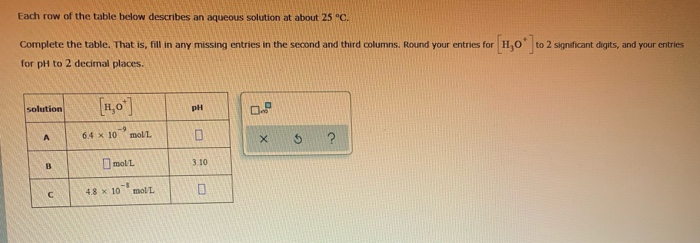Each row of the table below describes an aqueous solution at about 25 °C. to 2 significant digits, and your entries Complete the table. That is, fill in any missing entries in the second and third columns. Round your entries for H,O for pH to 2 decimal places. solution 6.4 x 10 moll 2 moll 48 x 10 molla

• ### It wont accept these numbers bc theyre not the right sig figs. 11. 1.29*10^-4mol/L 6.82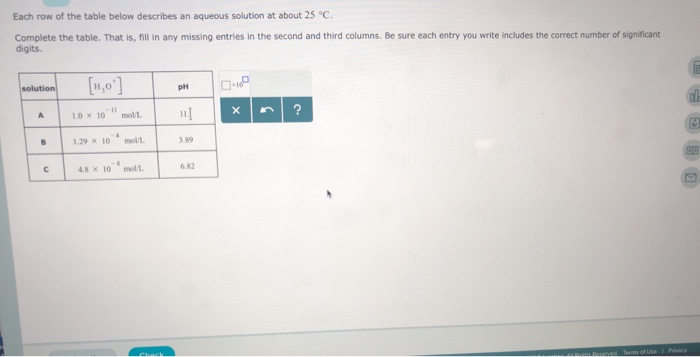it wont accept these numbers bc theyre not the right sig figs. 11. 1.29*10^-4mol/L 6.82 Each row of the table below describes an aqueous solution at about 25 °C Complete the table. That is, fill in any missing entries in the second and third columns. Be sure each entry you write includes the correct number of significant digits | □.ip pH ,-11 molL 1.0 × 10 B 1.29 x 10mol 3.89 6.82 Each row of the table below describes an...

• ### Each row of the table below describes an aqueous solution at 25 °C. The second column...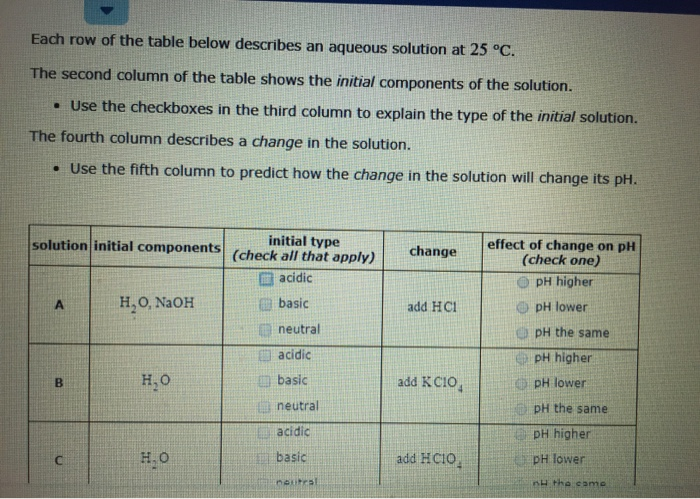Each row of the table below describes an aqueous solution at 25 °C. The second column of the table shows the initial components of the solution. • Use the checkboxes in the third column to explain the type of the initial solution. The fourth column describes a change in the solution. • Use the fifth column to predict how the change in the solution will change its pH. change solution initial components (check all that apply) initial type acidic H,...

• ### Each row of the table below describes an aqueous solution at 25 °C. The second column...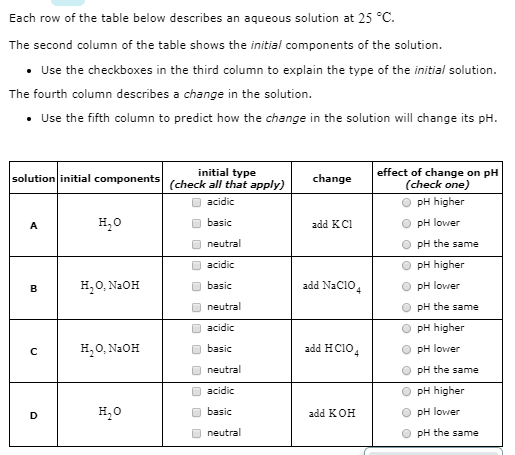Each row of the table below describes an aqueous solution at 25 °C. The second column of the table shows the initial components of the solution. • Use the checkboxes in the third column to explain the type of the initial solution. The fourth column describes a change in the solution. • Use the fifth column to predict how the change in the solution will change its pH. solution initial components change initial type (check all that apply) acidic effect...

• ### Each row of the table below describes an aqueous solution at 25 °C The second column...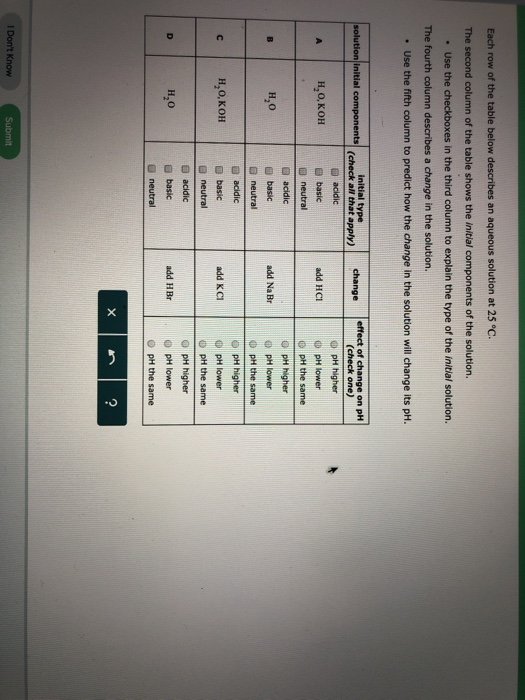Each row of the table below describes an aqueous solution at 25 °C The second column of the table shows the initial components of the solution. . Use the checkboxes in the third column to explain the type of the initial solution. The fourth column describes a change in the solution. Use the fifth column to predict how the change in the solution will change its pH. solution initial applychange effect of change on pH (check one) PH higher add...

• ### Each row of the table below describes an aqueous solution at 25°C . The second column...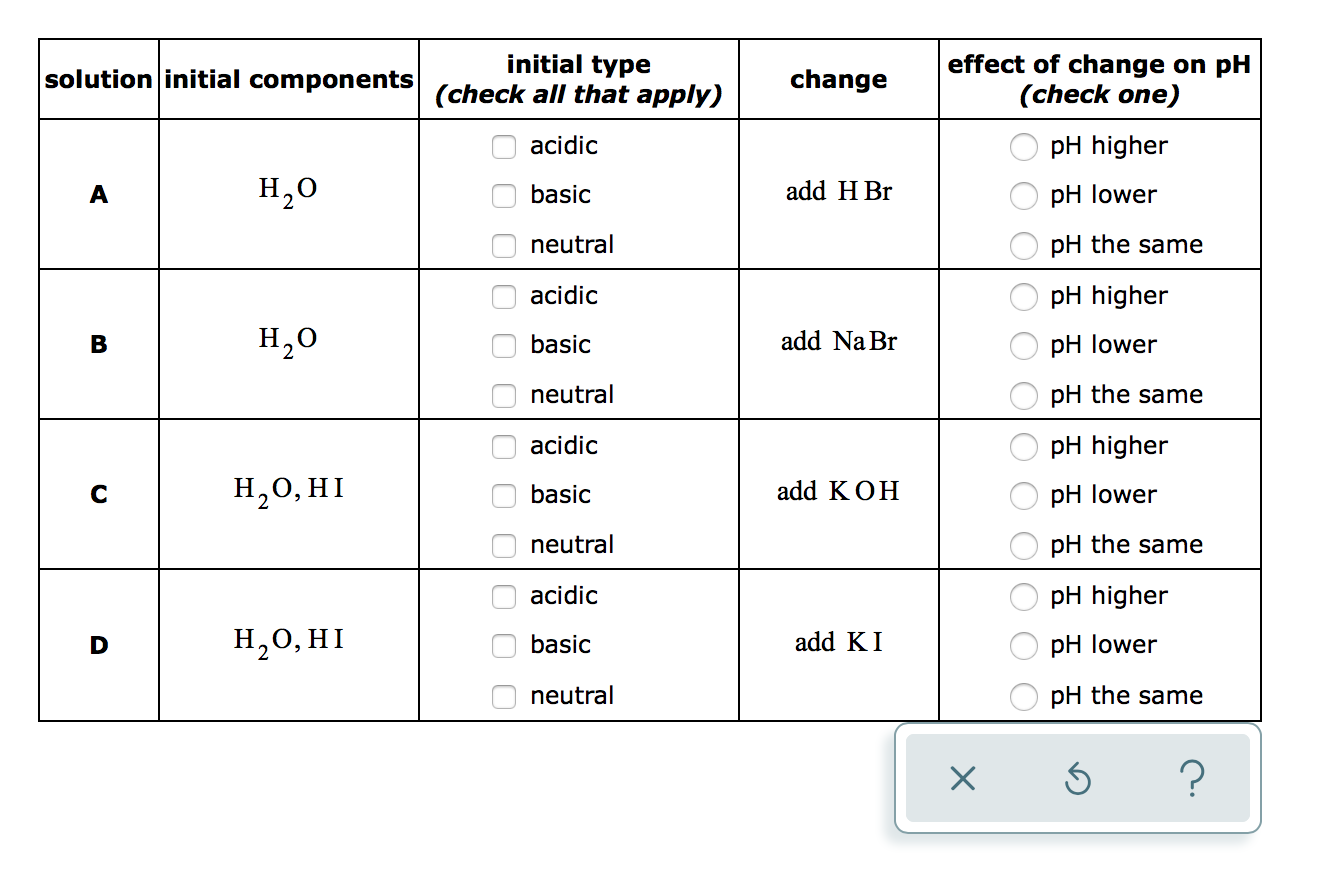Each row of the table below describes an aqueous solution at 25°C . The second column of the table shows the initial components of the solution. Use the checkboxes in the third column to explain the type of the initial solution. The fourth column describes a change in the solution. Use the fifth column to predict how the change in the solution will change its pH. initial type solution initial components cah mponents (check all that apply) change effect of...

• ### Each row of the table below describes an aqueous solution at 25°C. The second column of...

Each row of the table below describes an aqueous solution at 25°C. The second column of the table shows the initial components of the solution. Use the checkboxes in the third column to explain the type of the initial solution. The fourth column describes a change in the solution. Use the fifth column to predict how the change in the solution will change its pH. solution initial components initial type (check all that apply) change effect of change on pH...

• ### Each row of the table below describes an aqueous solution at 25 °C. The second column...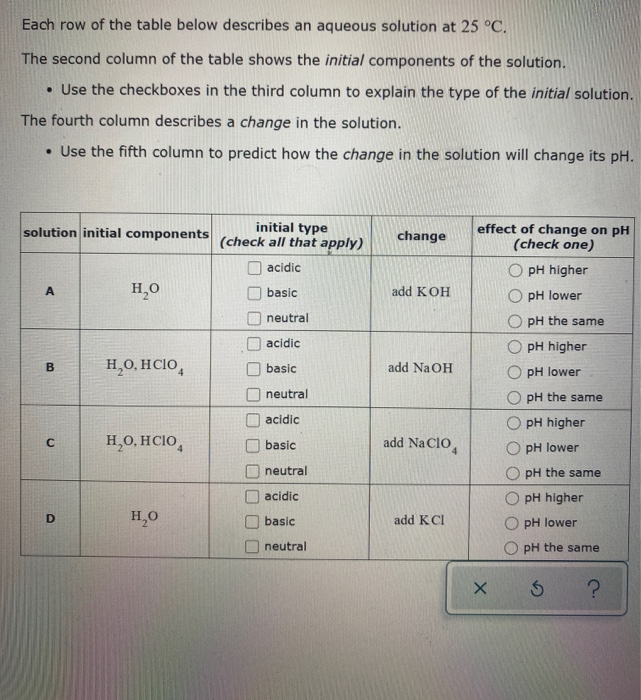Each row of the table below describes an aqueous solution at 25 °C. The second column of the table shows the initial components of the solution. • Use the checkboxes in the third column to explain the type of the initial solution. The fourth column describes a change in the solution. • Use the fifth column to predict how the change in the solution will change its pH. solution initial components change initial type (check all that apply) acidic A...

• ### Each row of the table below describes an aqueous solution at 25 °C. The second column...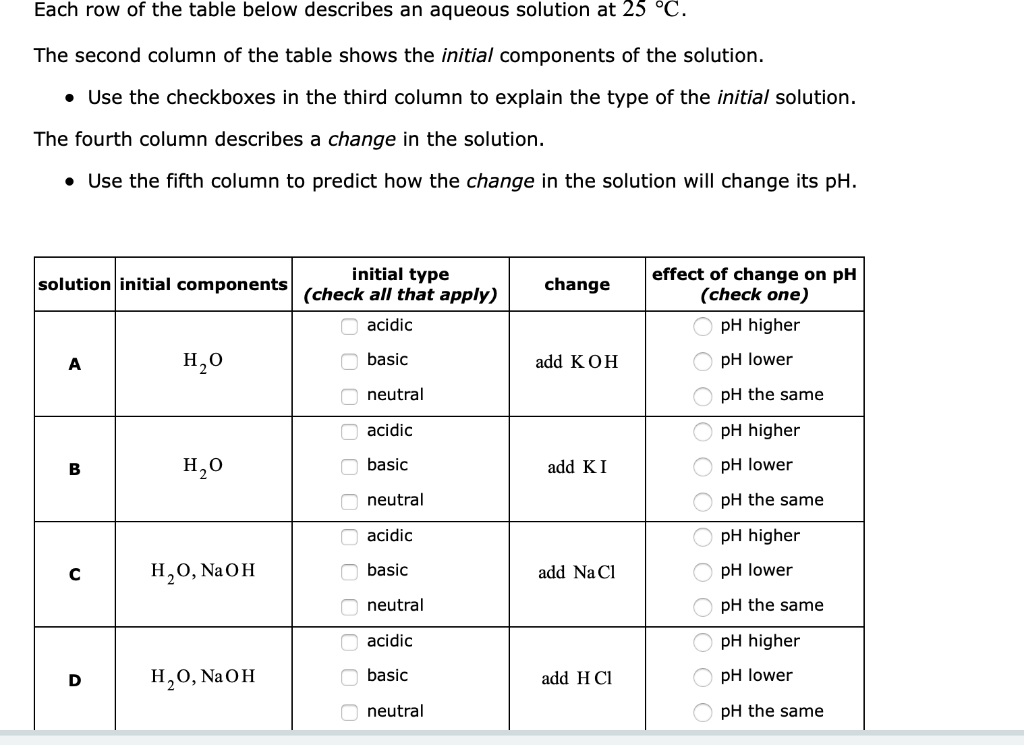Each row of the table below describes an aqueous solution at 25 °C. The second column of the table shows the initial components of the solution. • Use the checkboxes in the third column to explain the type of the initial solution. The fourth column describes a change in the solution. • Use the fifth column to predict how the change in the solution will change its pH. change solution initial components (check all that apply) initial type acidic H2O...

• ### Making qualitative estimates of pH change: Each row of the table below describes an aqueous solution...Making qualitative estimates of pH change: Each row of the table below describes an aqueous solution at 25°C. The second column of the table shows the initial components of the solution. Use the checkboxes in the third column to explain the type of the initial solution. The fourth column describes a change in the solution. Use the fifth column to predict how the change in the solution will change its pH. solution initial components initial type (check all that apply)...